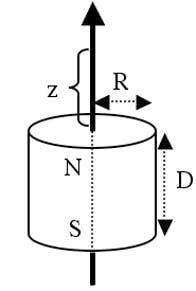# Formula to calculate the pull force between a magnet and a steel plate

Summary:
need a simple mathematical formula to calculate the pull strength of a neodymium magnet
i have a neobydium magnet of grade n40 , the diameter of magnet is 15mm and thickness is 10 mm ,
now i want to calculate the pull force between this magnet and a steel plate when there is no gap between them ;
& in second case i want to calculate the pull force when i put a 0.15mm copper plate between magnet and steel plate ,
does introducing copper plate reduce the pull strength , and if yes how ?
can you get me a formula by which i can calculate the pull strenth of magnet in the above mentioned conditions
i need a simple mathematical formula

I think a good, off-the-top-of-my-head, approximation of the attraction of a magnet to a metal surface would be:
A = c/d^2; were A= attraction force, c = coupling constant for a any given combination of magnet type and metal surface type (to be determined by experimentation). d = the distance between the magnet and the metal surface. You will need to select the desired units (metric, imperial etc.) and add additional factors to equate the terms.

https://www.kjmagnetics.com/calculator.aspSimple formula that is accurate? No.
This is an overview with fill in the blank calculator pages. Following links does provide clues as to why it is not simple.

i know this website but my boss wants a mathematical formula that can give the pull force and i cant find it anywhere on internet

•anorlunda
As others have mentioned, calculating this force is not so easy, the flux density of the magnet for example will vary according to the load line and the introduced reluctance from the steel.

An easy one though is the copper plate spacer, since PM's are a DC field, the copper with its relative permeability of ~1 simply acts as if it were an air gap.

An approximate calculation can be as follows:

1) calculate the magnetic flux density at distance z on the disc central axis:

B=Br/2*((D+z)/sqrt(R^2+(D+z)^2)-z/sqrt(R^2+z^2))

2) calculate the force

F=B^2*A/2/μo=B^2*A/(8*п)*10^7 [N]

B in T[Wb/m^2] ; A[area] in m^2Good morning, i hope you are having a great day. I would like to ask if you have the references for this formula. Thanks!

It seems to me the above link does not allow in 2021 to get the formula. However I got it only this way in 2019!